# Cosine-cubed function

(diff) ← Older revision | Latest revision (diff) | Newer revision → (diff)
This article is about a particular function from a subset of the real numbers to the real numbers. Information about the function, including its domain, range, and key data relating to graphing, differentiation, and integration, is presented in the article.
View a complete list of particular functions on this wiki
For functions involving angles (trigonometric functions, inverse trigonometric functions, etc.) we follow the convention that all angles are measured in radians. Thus, for instance, the angle of$90\,^\circ$ is measured as$\pi/2$.

## Definition

This function, denoted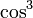$\cos^3$, is defined as the composite of the cube function and the cosine function. Explicitly, it is the function: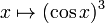$x \mapsto (\cos x)^3$.

## Key data

Item Value
default domain all real numbers, i.e., all of$\R$.
range the closed interval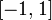$[-1,1]$, i.e.,$\{ y \mid -1 \le y \le 1 \}$.
period$2\pi$, i.e.,$360\,^\circ$
local maximum values and points of attainment All local maximum values are equal to 1, and they are attained at all points of the form$2n\pi$ where$n$ varies over integers.
local minimum values and points of attainment All local minimum values are equal to -1, and they are attained at all points of the form$(2n+1)\pi$ where$n$ varies over integers.
points of inflection (both coordinates) All points of the form$(n\pi + \pi/2,0)$ and Fill this in later
derivative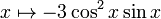$x \mapsto -3\cos^2x\sin x$
second derivative$x \mapsto 6\cos x \sin^2 x - 3 \cos^3x = 6\cos x - 9 \cos^3x$
antiderivative$\! \sin x - \frac{\sin^3x}{3} + C$
power series$\sum_{k=0}^\infty \frac{(-1)^k(3 + 3^{2k})x^{2k}}{4 \cdot (2k)!} = 1 - \frac{3x^2}{2} + \frac{7x^4}{8} - \dots$
converges everywhere to the function, also the Taylor series.

## Graph

Below is a basic picture of the graph, with the domain restricted to the interval$[-2\pi,2\pi]$:

A more close-up view, restricted to the interval$[-\pi,\pi]$, is below:

The thick red dots represent points of inflection and the thick black dots represent local extreme values.

## Integration

### First antiderivative: standard method

We rewrite$\cos^3 x = \cos^2x \cos x = (1 - \sin^2x)\cos x$ and then use integration by u-substitution, setting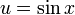$u = \sin x$. Explicitly: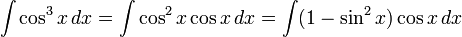$\! \int \cos^3 x \, dx = \int \cos^2x \cos x \, dx = \int (1 - \sin^2x) \cos x \, dx$

Setting$u = \sin x$, we get:$\int (1 - u^2) \, du$

This gives us:$u - \frac{u^3}{3} + C$

Plugging back$u = \sin x$, we get: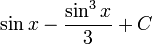$\sin x - \frac{\sin^3x}{3} + C$

### First antiderivative: using triple angle formula

An alternative method of integration is to use the identity:$\! \cos^3x = \frac{3\cos x + \cos(3x)}{4}$

We thus get: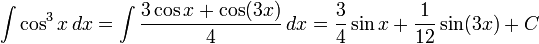$\int \cos^3x \, dx = \int \frac{3\cos x + \cos(3x)}{4} \, dx = \frac{3}{4} \sin x + \frac{1}{12}\sin(3x) + C$

Although this answer appears different from the other one, the two antiderivatives are in fact equal, as we can see by plugging in$\sin(3x) = 3\sin x - 4\sin^3x$ into the above antiderivative expression.

### Repeated antidifferentiation

The antiderivative of$\cos^3$ involves sin^3 and sin, which can both be antidifferentiated, with the new antiderivative involving$\cos^3$ and cos. The procedure can thus be repeated ad infinitum.

## Power series and Taylor series

### Computation of power series

We can use the identity: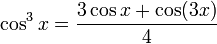$\cos^3x = \frac{3\cos x + \cos(3x)}{4}$

We have the power series:$\! \cos x = 1 - \frac{x^2}{2!} + \frac{x^4}{4!} - \dots = \sum_{k=0}^\infty \frac{(-1)^kx^{2k}}{(2k)!}$

Thus, we get: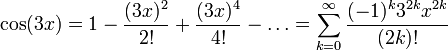$\! \cos(3x) = 1 - \frac{(3x)^2}{2!} + \frac{(3x)^4}{4!} - \dots = \sum_{k=0}^\infty \frac{(-1)^k3^{2k}x^{2k}}{(2k)!}$

Combining, we get: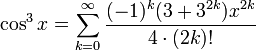$\! \cos^3x = \sum_{k=0}^\infty \frac{(-1)^k(3 + 3^{2k})x^{2k}}{4 \cdot (2k)!}$

The first few terms are:$\! \cos^3x = 1 - \frac{3x^2}{2} + \frac{7x^4}{8} - \dots$